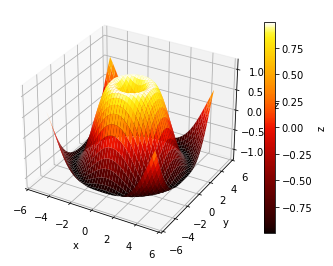# Surface 3d#

URL: http://matplotlib.org/examples/mplot3d/surface3d_demo.html

Most examples work across multiple plotting backends, this example is also available for:

```import numpy as np
import holoviews as hv
hv.extension('matplotlib')
```## Define data#

```# Make data.
X = np.arange(-5, 5, 0.25)
Y = np.arange(-5, 5, 0.25)
X, Y = np.meshgrid(X, Y)
R = np.sqrt(X**2 + Y**2)
Z = np.sin(R)

surface = hv.Surface(Z, bounds=(-5, -5, 5, 5))
```

## Plot#

```surface.opts(colorbar=True)
```This web page was generated from a Jupyter notebook and not all interactivity will work on this website. Right click to download and run locally for full Python-backed interactivity.